×

Search anything:

# Vector of Pair in C++

#### Software Engineering C++Get this book -> Problems on Array: For Interviews and Competitive Programming

In this article, we have covered the idea of Vector of Pair in C++ with code examples along with basics of Pair and vector in C++.

1. Basic of Pair in C++
2. Basic of Vector in C++
3. Vector of pairs in C++

Let us get started with Vector of Pair in C++.

# Basic of Pair in C++

Pair is a container that stores two data elements in it. It is not necessary that the two values or data elements have to be of the same data type.

Syntax: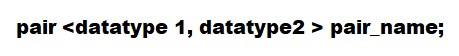The first value given in above pair could be accessed by using pair_name.first similarly, the second value could be accessed using pair_name.second.

Code:

``````#include <iostream>
using namespace std;
int main()
{
pair<int ,string> p; // Declaring a pair named p
p.first=1;  // Accessing the first element
p.second="Hello"; // Accessing the second element
cout<<"First element is: "<<p.first<<endl;
cout<<""Second element is: ""<<p.second;
return 0;
}
``````

Output: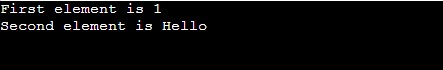# Basic of Vector in C++

Vectors are containers that can store multiple data elements that can change in size. The data elements in vectors are of the same data type. Vector is a dynamic array where the size of the array can changed. Vectors are stored in the stack but the elements of this Vector are stored in the heap memory.

Syntax: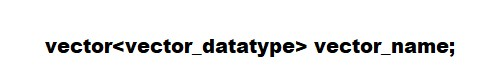There are some functions that are used in vectors to iterate,access elements,modify elements,to check the capacity of the vectors.

Iterators:

1.begin(): This function returns an iterator pointing to the first element of vector.
2.end(): This function return an iterator pointing to the element behind the last element of the vector.
3.cbegin(): This function returns a constant iterator pointing to the first element of vector.
4.cend(): This function returns an iterator pointing to the element behind last element of vector.
5.rbegin():This function returns a reverse iterator pointing to the last element of vector.
6.rend():This function returns a reverse iterator pointing to the preceding element before the first element of vector.

Accessing the elements:

1.at(j)-Returns a reference to element at position 'j'.
2.front()-Returns a reference to first element.
3.back()- Returns a reference to last element;
4.a.operator()[j]:Returns a reference to element at position 'j'

Capacity:

1.size() – Returns the number of elements in the vector.
2.max_size() – Returns the maximum number of elements that the vector can hold.
3.capacity() – Returns the size of the storage space currently allocated to the 4.vector expressed as number of elements.
5.resize(n) – Resizes the container so that it contains ‘n’ elements.
6.empty() – Returns whether the container is empty.

Modifiers:

1.push_back(): The function inserts the elements into a vector from the back.
2.assign(): It assigns a new value to the vector elements by replacing old ones.
3.pop_back(): The pop_back() function is used to pop or remove the last elements from a vector.
4.insert(): This function inserts new elements before the position specified by the iterator.
5.erase(): This function is used to remove elements from a container from the specified position or range.
6.swap(): This function is used to swap the contents of one vector with another vector of the same type. Sizes may differ.
7.clear(): This function is used to remove all the elements of the vector container

Code:

``````#include <iostream>
#include <vector>
using namespace std;

int main()
{
vector <int> a; // Declaring a vector

for(int i = 0; i<=5;i++)
{
a.push_back(i);
}
for(int i = 0; i<a.size();i++)
{
cout<<a[i]<<endl;
}
}

``````

Output: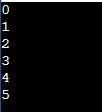# Vector of pairs in C++

Vector of Pairs is a dynamic array filled with pairs instead of any primitive data type. Vector of pairs are no different from vectors when it comes to declaration and accessing the pairs.

How do I declare a vector of pair? :
Ans: To declare a vector of pairs we can use the syntax : vector<pair<string,int>> a;

How can I add a pair to an existing vector of pairs? :
Ans: To add a pair to an existing vector of pairs we can use the statement below: a.push_back(make_pair("ABC",15));
or
a.emplace_back("ABC",15);
push_back function helps in adding the elements to a vector, make_pair function converts the parameters into pairs.

How to delete a pair from a vector?:
Ans: We can use a.pop_back(); to delete the last pair,
or
We can use a.erase(a.begin() + i); to delete from a specified position 'i'.

Code to add and delete pairs from vectors:

``````#include <iostream>
#include <vector>
using namespace std;

int main()
{
vector <pair<int,int>> vec={
{1,2},{3,4},{5,6}
};//Initializing a vector of pairs

vec.push_back(make_pair(7,8));//Adding a pair to the vector
cout<<"Vector contains pairs: ";
for(int i =0;i<vec.size();i++)
{
cout<<"("<<vec[i].first<<","<<vec[i].second<<")"<<" ";
}
cout<<endl;
vec.erase(vec.begin()+1);//Deleting vector at position i
cout<<"Vector after deletion: ";
for(int i =0;i<vec.size();i++)
{
cout<<"("<<vec[i].first<<","<<vec[i].second<<")"<<" ";
}
return 0;
}
``````

Output: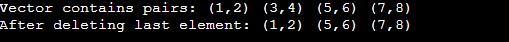Performance Of Vectors:

Vectors are quite similar to arrays when it comes to its functioning, despite that vectors are comparatively faster to arrays. Vectors are better when used for frequent insertion and deletion of elements, Arrays are quite better when it comes to frequent accessing of elements. In terms of space, vectors occupy more space than arrays.

• Random access - constant 𝓞(1)
• Insertion or removal of elements at the end - amortized constant 𝓞(1)
• Insertion or removal of elements - linear in the distance to the end of the vector 𝓞(n)

Applications:

• Vectors are dynamic arrays, that means the size of the vector changes after every insertion or deletion of data elements.Vectors can be very helpful in scenarios where there is a frequent change of data elements.

• In real time , Vectors are quite helpful in Computer vision, Artificial Intelligence, etc where the data is constantly being stored and being utilized for decision making.In this case the size of the data being interpreted is not fixed.

• Vectors are quite suitable for any applications making use of grid representation.
FAQs

• Which function inserts elements at a specific position in vectors?

Answer: insert() function helps in inserting elements at a specified position in vectors.

• How can I delete all the elements of a vector?

Answer: clear() function can be used to delete all elements in a vector.

• How to check the number of elements present in a vector?

Answer: size() function can be used to find the size of elements.

• How can I access a vector?

Answer: A vector can be accessed by either indexing it directly using reference operator or we can make use of at() function with some position passed as a parameter. To access the first element of vector we can use front() and back() can be used for the last element.

With this article at OpenGenus, you must have the complete idea of Vector of Pairs in C++.

#### Anubhav Tewari

Anubhav Tewari has been a Machine Learning Developer, Intern at OpenGenus.

Vector of Pair in C++